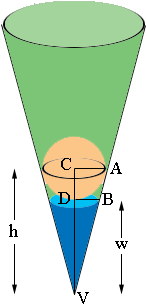SEARCH HOMEMath Central Quandaries & QueriesQuestion from Freddie, a student: A ball of diameter 20cm rests in a conical container whose angle with the slant height and the vertical axis is 25degrees. if water is poured into the container just enough to touch the bottom of the ball, find the quantity of water in the container.Freddie,You know that |AC| = 20 cm and that the measure of the angle CVA is 25o. Hence you can use the tangent function to find |CV| = h cm. Then you know w = h - 10 cm. Triangles ACV and BDV are similar and that gives you enough information to find the volume of water.

PennyMath Central is supported by the University of Regina and The Pacific Institute for the Mathematical Sciences.